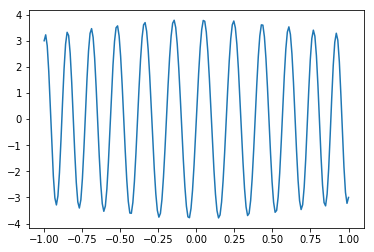# Finite Differences for Boundary Value Problems¶

In :
import numpy as np
import matplotlib.pyplot as pt

import scipy.sparse as sps


We'll solve

$u''+1000(1+x^2)u=0$ on $(-1,1)$

with $u(-1)=3$ and $u(1)=-3$.

In :
#n = 9
n = 200

mesh = np.linspace(-1, 1, n)
h = mesh - mesh


Use sps.diags(values, offsets=..., shape=(n, n)) to make a centered difference matrix.

In :
A = sps.diags(
[1,-2,1],
offsets=[-1,0,1],
shape=(n, n))

if n < 10:
print(A.todense())


Create second_deriv as a matrix to apply the second derivative. Can only do that for the interior points!

• change shape and offsets
• Take h into account
In :
second_deriv = sps.diags(
[1,-2,1],
offsets=np.array([-1,0,1])+1,
shape=(n-2, n))/h**2

if n < 10:
print(second_deriv.todense())


Make a matrix for the lower-order term.

In :
factor = sps.diags(
[1000*(1 + mesh[1:]**2)],
offsets=,
shape=(n-2, n))

if n < 10:
print(mesh[1:-1])
print()
print(factor.todense())


Build the matrix for the interior:

In :
A_int = second_deriv+factor

if n < 10:
print(A_int.todense())


Glue on the rows for the boundary conditions:

In :
A = sps.vstack([
sps.coo_matrix((, (,)), shape=(1, n)),
A_int,
sps.coo_matrix((, (,[n-1])), shape=(1, n)),
])
A = sps.csr_matrix(A)

if n < 10:
print(A.todense())


Next, assemble the right-hand side as rhs:

Pay special attention to the boundary conditions. What entries of rhs do they correspond to?

In :
rhs = np.zeros(n)
rhs = 3
rhs[-1] = -3


To wrap up, solve and plot:

In :
import scipy.sparse.linalg as sla

sol = sla.spsolve(A, rhs)

In :
pt.plot(mesh, sol)

Out:
[<matplotlib.lines.Line2D at 0x7fee957500f0>]In [ ]:


In [ ]: